# Experimental Certification of Random Numbers via Quantum Contextuality

### Subjects

• A Corrigendum to this article was published on 02 February 2018

## Abstract

The intrinsic unpredictability of measurements in quantum mechanics can be used to produce genuine randomness. Here, we demonstrate a random number generator where the randomness is certified by quantum contextuality in connection with the Kochen-Specker theorem. In particular, we generate random numbers from measurements on a single trapped ion with three internal levels and certify the generated randomness by showing a bound on the minimum entropy through observation of violation of the Klyachko-Can-Binicioglu-Shumovsky (KCBS) inequality. Concerning the test of the KCBS inequality, we close the detection efficiency loophole for the first time and make it relatively immune to the compatibility loophole. In our experiment, we generate 1 × 105 random numbers that are guaranteed to have 5.2 × 104 bits of minimum entropy with a 99% confidence level.

## Introduction

Random number generation is important for many applications1,2. For cryptographic applications, random numbers should have good unpredictability in order to be secure under attack by the adversaries3. Genuine random numbers can never be generated by a classical device because any classical device bears in principle a deterministic description. Quantum mechanics, on the other hand, has intrinsic randomness and thus can be explored to construct a genuine random number generator. There have been many demonstrations of random number generators based on quantum principles4,5,6,7,8,9,10,11,12,13,14.

Self-certified random number generation is an advance made recently, where the randomness is guaranteed by violation of certain fundamental inequalities14,15,16. In particular, it was proposed in Refs. 14,15 that through violation of the Clauser-Horn-Shimony-Holt (CHSH) inequality, one can certify the generated random numbers in a device-independent fashion that is secure against the adversaries who have only classical side information17. The first proof-of-principle experiment for this scheme has been recently demonstrated14.

We consider here a scenario where the provider of the device is assumed to be honest. However, we still need to physically certify that the random numbers are generated due to the intrinsic uncertainty of quantum mechanics instead of some uncontrolled classical noise process in the device. In this case, we can use quantum contextuality manifested through the violation of certain Kochen-Specker (KS) inequality to certify the generated random numbers18,19. Quantum contextuality is a basic property of quantum mechanics, where the measurement outcomes depend on the specific context of the measurements20,21. Quantum contextuality would be revealed by violations of some KS inequalities and such violations can be observed even in a single indivisible system without any entanglement22,23,24,25,26,27. Because there is no need of entanglement, a certification scheme of random numbers based on the KS theorem can significantly simplify the experimental requirement and generate certified random numbers with a much higher speed18. A proof-of-principle experimental implementation of this idea has been reported with a photonic system quite recently18.

A particular type of the KS inequality, the Klyachko-Can-Binicioglu-Shumovsky (KCBS) inequality22, is convenient for certification of random numbers. Violation of the KCBS inequality has been observed before in a single-photonic system23. For experimental test of the KCBS inequality, there are two possible loopholes: the detection efficiency loophole if the detectors only register a subset of data due to their inefficiency and the compatibility loophole, which occurs if additional assumptions are required to guarantee that the observables with simultaneous assignment of values in the KCBS inequality are compatible with each other and remain identical when their measurement contexts change. The test of the KCBS inequality with the photonic system is immune to the compatibility loophole23, however, it requires the fair-sampling assumption due to the low photon detection efficiency and thus subject to the detection efficiency loophole.

In this paper, we report a random number generator certified by quantum contextuality with a single trapped ion, which allows us to close the detection efficiency loophole for the first time for the KCBS inequality. For the compatibility, we follow basically the same configurations as in Ref. 23, where errors in compatibible measurement settings only reduce the amount of the violations. Even with experimental noise and imperfections, we get significant violations of the KCBS inequality, which lead to lower bounds the minimum entropy of the generated random string. Compared to the experimental certification based on the CHSH inequality14, the generation rate of random numbers is increased by about four orders of magnitudes in our experiment, which is important for practical applications.

The paper is organized as follows. First, we introduce the KCBS inequality and show the experimental violation of this inequality. Then, we introduce the relation between the violation of the KCBS inequality and the minimum entropy of the generated random string for the case of an honest provider and compare the theoretical prediction with our experimental observation. The generated random bits are tested under uniform or biased choice of measurement settings. We conclude the paper by summarizing the results and discussing further improvements of our random number generation scheme.

## Results

### The KCBS inequality

The Kochen-Specker theorem states that the results of quantum mechanics cannot be fully explained by non-contextual classical theories which assume that the measurement outcomes of a physical system are predetermined and independent of their own and other simultaneous compatible measurements20,21. The KCBS inequality illustrates the conflict between quantum mechanics and non-contextual classical theory in the simplest possible system with the Hilbert space dimension d = 322.

The KCBS inequality is connected with the following simple algebraic equation.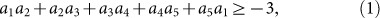where the value of ai is either 1 or −1. If the values of the observables are predetermined, the average of the left hand of the above equation should be no less than −3, leading to the following inequality: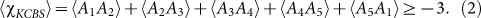In quantum mechanics, however, the outcomes of Ai do not have predetermined values, which allows violation of the KCBS inequality (2) for a specific state |ψ0〉 in systems with d ≥ 3. In the case of d = 3, we denote the bases by |1〉, |2〉 and |3〉 and the observable Ai, represented by Ai = 1 – 2 |vi〉 〈vi|, is the projector on the axis |vi〉. The maximal violation of the KCBS inequality (2) is achieved for the state along the symmetric axis of the pentagram shown in Fig. 1(a). Here |v1〉 = |1〉, |v2〉 = |2〉, |v3〉 = R1 (γ, 0) |v1〉, |v4〉 = R2 (γ, 0) |v2〉, |v5〉 = R1 (γ, 0) |v3〉 and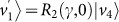, where γ = 51.83° and R1,2 denote the rotation operations between |1〉 to |3〉 and between |2〉 to |3〉, respectively. Maximal violation the KCBS inequality is achieved under the state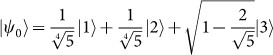(2), with the corresponding value.

Figure 1(b) shows the scheme for preparation of the initial state |ψ0〉 starting from the basis state |3〉 and Fig. 1(c)–(g) describe the implementation of the measurement configurations along the five axes. To ensure context independence, we emphasize that the measurement configuration of Ai remains the same when it is measured with either Ai–1 or Ai+1 (let A0A5, A6A1). For example, the scheme for the measurement A2 is exactly the same in the first [Fig. 1(c)] and the second stage [Fig. 1(d)]. To move to the second configuration, we perform a rotation between the states |1〉 and |3〉, which does not influence the state |2〉 that corresponds to the observable A2. Only the observable related to the state |1〉 is changed from A1 to A3.

The configuration for the measurement of A1 in Fig. 1(c) is not the same as that in Fig. 1(g), which is therefore denoted by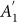. If A1 and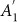are not identical, it is possible to violate the inequality (3) even in classical theory. To solve this problem, similarly to Ref. 23, we use a new inequality that includes the observablewith the formNote that the inequality (3) becomes the original KCBS inequality (2) when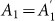. Therefore, the difference between two measurements decrease the violation that can be obtained in the experiments23. Another possible way out is to introduce an empirical parameter to upper bounds the violation of compatibility, which would be similar in spirit to a recent work where a parameter in introduced to bound violation of the locality loophole for test of the Bell inequality28. Any imperfection in the initial state preparation or final measurements only leads to a reduction of violation of the KCBS inequality, so a significant violation of this inequality guarantees that the randomness comes from the quantum origin instead of a classical noise process.

### Experimental violation of the KCBS inequality

The violation of the KCBS inequality have been observed with single photons18,23, however, those experiments are subject to the detection efficiency loophole. Here, we present the experimental violation of the KCBS inequality in a single trapped ion. Because of the high detection efficiency for the trapped ion, we close the detection efficiency loophole for the first time for this inequality.

We perform the test of the KCBS inequalities (2) with a single trapped 171Yb+ ion in a four-rod radio-frequency trap26,29. The qubit states are represented by the two internal levels in the S1/2 ground-state manifold, with |F = 1, mF = 0〉 ≡ |↑〉 and |F = 0, mF = 0〉 ≡ |↓〉. The transition frequency between |↑〉 to |↓〉 is ωHF = (2π)12642.821 MHz, determined by the hyperfine interaction.

The procedure of the experiment consists of Doppler cooling, initialization, coherent operation and detection (see the Method Section). The initial state preparation and the measurement configurations are shown in Fig. 1(b)-(g) and they are realized by two microwaves with the frequencies ω1 and ω2, which produce Rabi oscillations R11, ϕ1) and R22, ϕ2) between |1〉 to |2〉 and between |1〉 to |3〉, respectively. Here, θ1,2 and ϕ1,2 are controlled by the duration and phase of the microwaves. R11, ϕ1) and R22, ϕ2) are have the following explicit forms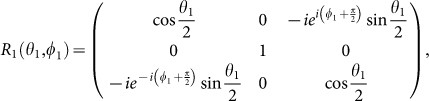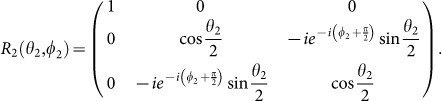For experimental convenience, we transform the observable Ai to Vi = (1 − Ai)/2, which is assigned to value vi = 0 when photons are detected or vi = 1 when no photons are detected. With Vi, the KCBS inequality (3) is rewritten as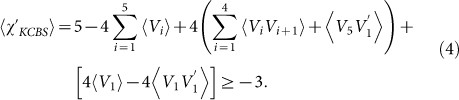We obtain 〈Vi〉 by mapping the axis vi to the state |3〉 and then measuring the probability P|3〉 (vi = 1) = 〈Vi〉 (Fig. 2(b)). For simplicity, let P|3〉 = P. The correlation terms 〈ViVi+1〉 are obtained by sequential measurements depicted in Fig. 2(c). First, we transfer Vi on the state |3〉 and apply the standard fluorescence detection scheme. If we detect photons, the state should not be |3〉 and we assign vi = 0 to the observable Vi, where the outcome of the correlation term ViVj vanishes and no further measurements are needed. If we detect no photons, we assign vi = 1 to the Vi. Then, we apply the swapping microwave π-pulse that converts Vj to |3〉 before another round of fluorescence detection. If we observe photons, vj = 0 and if no photons, vj = 1. We asign the value 1 to the correlation term ViVj only when we detect no photons for both rounds of measurements. We obtain the average of the correlation term 〈ViVj〉 = P (vi = vi+1 = 1) by repeating the same experimental sequence many times26.

The expectation value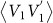is obtained by the scheme shown in Fig. 2(d). If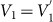ideally, the correlation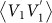shoud be same to 〈V1〉 since V1 is projection operator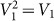. The state |1〉 at the beginning of Fig. 1(g) corresponds to the observable V1, which is exactly the same configuration as in Fig. 1(c). Therefore, if photons are detected (v1 = 0) or not detected (v1 = 1) at the place where V1 would be measured, photons should be observed (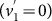) or not be observed (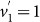) for the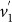shown in Fig. 2 (d). After repeating the sequence of Fig. 2(d), we acquire the probability that no photons are measured (), which gives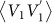by definition.

We randomly choose one of the five configurations (c)–(g) of Fig. 1 based on computer generated random numbers and perform the sequential measurements. We change the order of sequential measurements ( ViVi+1 or Vi+1Vi) with equal probability. We occasionally check the overlap of V1 and. We repeat the sequences 1 × 105 times and observe 〈χKCBS〉 = 3.852(0.030), which violates the extended KCBS inequality (3,4) by 31 σ. The detailed results of the measurements are summarized in Table 1. We emphasize that our result of the violation cannot be explained by any non-contextual classical theory which does not exploit the compatibility loophole (the detection loophole is closed in our experiment). In other words, any classical part of the system such as technical noise, imperfections and/or unexpected changes of control parameters can not produce the violation. Therefore, as long as we observe the violation of the inequality, we can ensure that the outcomes of our measurements originate from quantum mechanics.

### The relation between violation of the KCBS inequality and the min-entropy

We establish the relation between violation of the KCBS inequality (2, 4) and randomness of the generated string from the experiment, similar to the photonic demonstration18. We focus on the scenario with an honest provider of the device17 rather than the extreme adversary scenario where the device has been produced by a malicious manufacturer. Even though we trust the device provider, we still need to ensure that the randomness of the generated sequence is caused by quantum uncertainty instead of technical noise17. For this purpose, we assume: (1) the system can be described by quantum theory; (2) the input at lth trial is chosen from a random process that is independent and uncorrelated from the system and its value is revealed to the system only at step l; (3) the outcomes of the corresponding pairs of measurements at step l are compatible (the measurement of one observable does not influence on the marginal distribution of the results of the other observable); (4) the adversary does not have any capability of controlling the inside of the system. The first and the second assumptions here are identical to those made in the certification scheme of Bell's inequality14. The third is the contextuality assumption that replaces the role of locality assumption for the Bell inequality. The fourth is an assumption about the honest provider17.

We consider five sets of measurement configurations S = {A1A2, A2A3, A3A4, A4A5, A5A1}, where Ai is the observable with the output ai = ±1 and compatible with Ai–1 and Ai+1. We can rewrite the KCBS inequality (2) as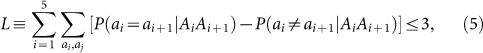where P (ai = ai+1|AiAi+1) or P (aiai+1|AiAi+1) is the probability that the output results are the same or different for a chosen measurement setting AiAi+1. Note that we change the sign of the inequality to make the derviation similar to that in Refs. 14,17,30. In our experiment, since we use the observable Vi (result vi = 0,1) intead of Ai and only distinguish the event of vi = vi+1 = 1 from others, the Eq. (5) is modified as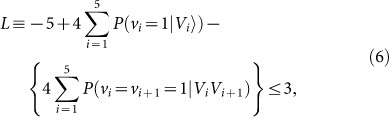where P (vi = 1|Vi) is the probability that the output result vi is 1 at a measurement setting Vi. The result of terms inside {··· } is ideally zero and non-zero positive value can be occurred by experimental errors or imperfections, which only reduces the amount of violation from the optimal. Therefore, we can conclude that the experimental violations of the inequality (6) arise from solely quantum mechanical origin not any classical mean.

In our relization, we estimate the violation of the inequality (6) by repeating the sequences n times and additional runs ncc of the comparibility check, the measurement setting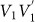. The estimation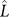of Eq. (5), obtained from the experimental data, is written as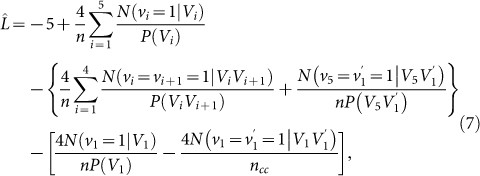where N (vi = 1|Vi) or N (vi = vi+1 = 1|ViVi+1) is the number of times that the outcome vi or vi and vi+1 is one under a measurement setting Vi or Vi and Vi+1, respectively. P (Vi) or P (ViVi+1) is the probability with which a measurement configuration Vi or Vi and Vi+1 is chosen. Note that positive result of terms inside {···} and [···] originates from the experimental flaws, which only reduces the amount of violation.

The randomness of a single generated bit vi from a measurement setting Vi can be characterized by the min-entropy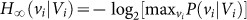, where P (vi|Vi) is the conditional probability of obtaining vi when the input setting Vi and the maximum is taken over all possible values of the output string. The theorem 1 of Ref. 17 shows that the min- entropy of the generated string after n trials is bounded by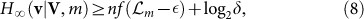where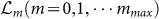is a series of KCBS violation thresholds with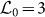the classical bound and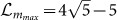the maximum violation and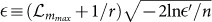, with r the smallest probability of input choices miniP (Vi). The parameterparameter denotes the closeness between the resulting distribution that characterize k successive uses of the device and another extended distribution that is well defined mathematically. The function f is found by semidefinite programming at various expectations L. Fig. 3 presents how the min-entropies are affected by the confidence levels,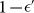and 1 − δ. When we set a high confidence level,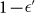, the bound on the min-entropy reduces as expected. Note that the certified min-entropy is only determined by measured value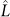and the choice of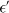, independent of experimental details.

### Random number results

We perform ten thousand trials to generate random bits as described in the previous section: [Experimental violation of the KCBS inequality]. At each trial, we choose one of the five measurement configurations shown in Fig. 1 (c)−(g) by computer-generated random numbers, perform the sequence composed of Doppler cooling, state initialization and rotations for the chosen configuration and finally record the existence of fluorescence (see Method section). As explained, we obtain a random bit, i.e., 1 (or 0) with fluorescence (or no fluorescence) for each trial. The sequence takes about 10 ms, mainly limited by the wave-form loading time to the pulse generator. Note that the random generator based on the CHSH inequality produced a random bit per several min.

Figure 4 shows the min-entropies of generated strings discussed in the previous section: [The relation between violation of the KCBS inequality and the min-entropy]. We produce a string of length 1 × 105 with uniform choices of the measurement settings, P (Vi) = 1/5. As shown in Table 1, we observe the expectation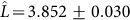, implying the min-entropy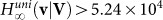with 99% confidence. Note that the other confidence level δ does not have any noticable influence on the bound of min-entropy. Here we used the thresholds of KCBS violations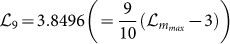.

Fig. 4 shows clearly the advantage of our certification scheme, i.e., we can guarantee the min-entropy of the generated random string by only monitoring the violation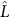independent of experimental details. Fig. 4(a) shows the accumulated behavior of the min-entropy as the number of experimental trials n increases. The solid lines show the theoretical linear increment of the minentropies and the slopes are determined by only the thresholds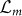. Due to drifts of experimental parameters, the violationsare fluctuating from one threshold to another, which accordingly introduces the changes to the min-entropy, accordingly. Fig. 4(b) shows details of the transient behavior of the generated random string. We monitor the violation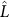for each batch of n = 1 × 104 trials and estimate the min-entropy in the batch. Fig. 4(b) reveals that the min-entropies are correlated to the violations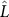and completely determined by the thresholds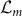at given confidence level 99%. Here, we we do not need massive random tests to ensure the amount actual random number in the generated string. The amount of min-entropy of our random numbers is guaranteed by the the measured violations, regardless of unexpected changes of experimental parameters.

We also generate random bits with a biased choice of measurement settings, where P (V1) = 1 − 4q, P (V2) = P (V3) = P (V4) = P (V5) = q and q = αn−1/2 with α = 6 and n = 105. We observe basically the same behavior of the min-entropy for the generated stream except for a slightly smaller bound due to the non-uniform setting. We get the min-entropy bound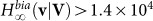from 1 × 105 rounds with violation of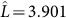. For the biased choice of measurement settings, the output entropy (1.35 × 104) exceeds the input entropy (1.14 × 104) and we obtain 2.1 × 103 net random bits. For the case of uniform measurement settings, we always need more initial randomness and thus cannot obtain net randomness. This is similar to the random number generation scheme with the CHSH inequality, where to generate net randomness, one always needs to consider nonuniform measurement settings.

Finally, we carry out a series of random tests (see Methods)31 to examine the quality of our random numbers obtained by collecting the outcomes of the first measurement in each trial. As expected, our generated random numbers passed all the tests. Fig. 5 shows the summary of the test results. Actually the real randomness of our generated strings is already certified by the KCBS inequality, which is a much stronger statement than claiming that the produced numbers pass all the random tests, since no random tests on finite strings should be considered complete.

## Discussion

In summary, we have demonstrated violations of the KCBS inequality using a single trapped ion, with the detection efficiency loophole closed for the first time. We use quantum contextuality to certify randomness of the measurement outcomes. The randomness of our device is ensured by observing violations of the inequality independent of experimental details. With our device, we already obtained a net output entropy. The device can generate random numbers with a higher speed, which is important for practical applications.

## Methods

### Experiment procedure

The experimental procedure consists of Doppler cooling, initialization, coherent operations and detection. After 1 ms Doppler cooling, the internal state of the ion is initialized to |3〉 by 3 μs standard optical pumping with efficiency 99.1%26. The states are coherently manipulated by the microwaves ω1 and ω2 that are resonant to the transitions between |1〉 and |3〉 and between |2〉 and |3〉, respectively. The quantum operations of the microwaves ω1 and ω2 are described by the rotation matrix R11, ϕ1) and R22, ϕ2), respectively. Here θ1, θ2 and ϕ1, ϕ2 are controlled by the duration and the phase of the applied microwaves. The 2 π times for both Rabi oscillations are adjusted to 29.5 μs, that is Ω1,2 = (2π) 33.9 kHz in frequency. The maximum probability of off-resonant excitation Ω2/(ω2 − ω1)2 is about 1.6 × 10−5, small enough to ensure independence of each Rabi oscillation. The standard fluorescent-detection method enables us to differentiate between one state versus the other two states of a qutrit. We observe on average 10 photons at 369.5 nm for the |1〉 or the |2〉 state and detect no photon for the |3〉 state. The state detection error rates for wrongly registering the state |3〉 and missing the state |3〉 are 0.9% and 1.9%, respectively, with the discrimination threshold nph = 1. As shown in Fig. 2(b), we transfer the information of obervable Ai (Aj) by π-pulse and apply the measurement sequence. Then we assign the value ai = 1 (aj = 1) on the obsevable Ai (Aj) when photons detected or ai = −1 (aj = −1) when no photons are detected. After repeating the same experimental procedures, we obtain the 〈Ai〉 (〈Aj〉). Here we emphasize that our setup is not subject to detection loophole and provide a value of the measurement at every trial.

### Random test

We apply the random tests that are appropriate for the size of our random numbers, which are ‘Frequency’, ‘Block Frequency’, Cumulative Sums (Cusums)’, ‘Runs’, ‘Longest-Run-of-Ones in a Block (LROB)’, ‘Rank’, ‘Discrete Fourier Transform Test (FTT)’, ‘Approximate Entropy (AE), ‘Serial.’ The p-values of all the test, which are the probabilities that an ideal random number generator would produce less random sequence than the tested one. Therefore, a p-value of 0 simply means that the tested sequence appears to be completely non-random, whereas a p-value of 1 implies that the sequence in test appears to be perfectly random. The p-values lie in the open interval (0, 1) and if p-value is larger than a significance level θ, we accept the sequence as random for the test. Typically θ is chosen to be in the range [0.0001, 0.01] and we set θ = 0.01. Note that we use Von-Neumann extractor for the output strings to make uniform distributions, which reduces the size of random numbers to one quarter. We also note that the random tests are different from guaranteeing the amount of min-entropy in the generated string. In other words, even the data could not pass the random tests but still have the quoted min-entropy.

## Change history

• ### 02 February 2018

Scientific Reports 3: Article number: 1627; published online: 09 April 2013; updated: 02 February 2018. The original version of this Article contained an error in the spelling of the author Yangchao Shen, which was incorrectly given as Shen Yangchao. This error has now been corrected in the PDF and HTML versions of the Article.

## References

1. Coddington, P. D. Analysis of random number generators using monte carlo simulation. Northeast Parallel Architecture Center Paper 14 (1994).

2. Gisin, N., Ribordy, G., Tittel, W. & Zbinden, H. Quantum cryptography. Rev. Mod. Phys. 74, 145 (2002).

3. Goldreich, O. Foundations of Cryptography (Cambridge University Press, Cambridge, UK, 2007).

4. Isida, M. & Ikeda, Y. Random number generator. Ann. Inst. Stat. Math. 8, 119–126 (1956).

5. Stefanov, A., Gisin, N., Guinnard, O., Guinnard, L. & Zbinden, H. Optical quantum random number generator. J. Mod. Opt. 47, 595–598 (2000).

6. Jennewein, T., Achleitner, U., Weihs, G., Weinfurter, H. & Zeilinger, A. A fast and compact quantum random number generator. Rev. Sci. Instrum. 71, 1675–1680 (2000).

7. Ma, H. Q., Xie, Y. J. & Wu, L. A. A random number generator based on quantum entangled photon pairs. Chin. Phys. Lett. 21, 1961–1964 (2004).

8. Kwon, O., Cho, Y.-W. & Kim, Y.-H. Quantum random number generator using photon-number path entanglement. Appl. Opt. 48, 1774–1778 (2009).

9. Qi, B., Chi, Y.-M., Lo, H.-K. & Qian, L. High-speed quantum random number generation by measuring phase noise of a single-mode laser. Opt. Lett. 35, 312–314 (2010).

10. Gabriel, C. et al. A generator for unique quantum random numbers based on vacuum states. Nature Photonics 4, 711–715 (2010).

11. Bustard, P. J. et al. Quantum random bit generation using stimulated raman scattering. Opt. Exp. 19, 25173–25180 (2011).

12. Symul, T., Assad, S. M. & Lamb, P. K. Real time demonstration of high bitrate quantum random number generation with coherent laser light. Appl. Phys. Lett. 98, 231103 (2011).

13. Fiorentino, M., Santori, C., Spillane, S. M. & Beausoleil, R. G. Secure self-calibrating quantum random-bit generator. Phys. Rev. A 75, 032334 (2007).

14. Pironio, S. et al. Random numbers certified by bell's theorem. Nature 464, 1021 (2010).

15. Colbeck, R. Quantum and relativistic protocols for secure multi-party computation. Ph.D. thesis, University of Cambridge (2007).

16. Vazirani, U. & Vidick, T. Certifiable quantum dice. Phil. Trans. R. Soc. A 370, 3432–3448 (2012).

17. Pironio, S. & Massar, S. Security of practical private randomness generation. Phys. Rev. A 87, 012336 (2013).

18. Deng, D. L. et al. Exploring quantum contextuality to generate true random numbers. arXiv:1301.5364 (2013).

19. Abbott, A. A., Calude, C. S., Conder, J. & Svozil, K. Kochen-specker theorem revisited and strong incomputability of quantum randomness. arXiv:1207.2029 (2012).

20. Kochen, S. & Specker, E. P. The problem of hidden variables in quantum mechanics. J. Math. Mech. 17, 59–87 (1967).

21. Bell, J. S. On the problem of hidden variables in quantum mechanics. Rev. Mod. Phys. 38, 447–452 (1966).

22. Klyachko, A. A., Can, M. A., Binicioğlu, S. & Shumovsky, A. S. Simple test for hidden variables in spin-1 systems. Phys. Rev. Lett. 101, 020403 (2008).

23. Lapkiewicz, R. et al. Experimental non-classicality of an indivisible quantum system. Nature 474, 490–493 (2011).

24. Yu, S. & Oh, C. H. State-independent proof of kochen-specker theorem with 13 rays. Phys. Rev. Lett. 108, 020403 (2012).

25. Zu, C. et al. State-independent experimental test of quantum contextuality in an indivisible system. Phys. Rev. Lett. 109, 150401 (2012).

26. Zhang, X. et al. State-independent experimental tests of quantum contextuality in a three dimensional system. Phys. Rev. Lett. 110, 070401 (2013).

27. Kong, X. et al. An experimental test of the non-classicality of quantum mechanics using an unmovable and indivisible system. arXiv:1210.0961 (2012).

28. Silman, J., Pironio, S. & Massar, S. Device-independent randomness generation in the presence of weak cross-talk. Phys. Rev. Lett. 110, 100504 (2013).

29. Olmschenk, S. et al. Manipulation and detection of a trapped Yb+ hyperfine qubit. Phys. Rev. A 76, 052314 (2007).

30. Fehr, S., Gelles, R. & Schaffner, C. Security and composability of randomness expansion from bell inequalities. Phys. Rev. A 87, 012335 (2013).

31. Rukhin, A. et al. A statistical test suite for random and pseudorandom number generators for cryptographic applications. NIST special publication 800–22, Rev. 1–a (2010).

## Acknowledgements

We thank Periklis Papakonstantiou, Dominik Scheder and Xiongfeng Ma for helpful discussion. This work was supported in part by the National Basic Research Program of China Grant 2011CBA00300, 2011CBA00301, 2011CBA00302, the National Natural Science Foundation of China Grant 61073174, 61033001, 61061130540. KK acknowledges the support from the Thousand Young Talents program.

## Author information

M.U., X.Z., J.Z. and Y.W. developed the experimental setup for the measurements. M.U., X.Z., J.Z., Y.W. and S.Y. carried out the measurements. M.U. analyzed the data and D.L.D., L.M.D. provided the theoretical support. K.K. supervised the experiment. All authors participated in writing the manuscript.

## Ethics declarations

### Competing interests

The authors declare no competing financial interests.

## Rights and permissions

Reprints and Permissions

Um, M., Zhang, X., Zhang, J. et al. Experimental Certification of Random Numbers via Quantum Contextuality. Sci Rep 3, 1627 (2013). https://doi.org/10.1038/srep01627

• Accepted:

• Published:

• ### Resource theory of contextuality

• Barbara Amaral

Philosophical Transactions of the Royal Society A: Mathematical, Physical and Engineering Sciences (2019)

• ### Parity oblivious d-level random access codes and class of noncontextuality inequalities

• Andris Ambainis
• , Manik Banik
• , Anubhav Chaturvedi
• , Dmitry Kravchenko
•  & Ashutosh Rai

Quantum Information Processing (2019)

• ### Characterizing and quantifying extended contextuality

• Barbara Amaral
•  & Cristhiano Duarte

Physical Review A (2019)

• ### Experimentally probing the algorithmic randomness and incomputability of quantum randomness

• Alastair A Abbott
• , Cristian S Calude
• , Michael J Dinneen
•  & Nan Huang

Physica Scripta (2019)

• ### Noncontextual Wirings

• Barbara Amaral